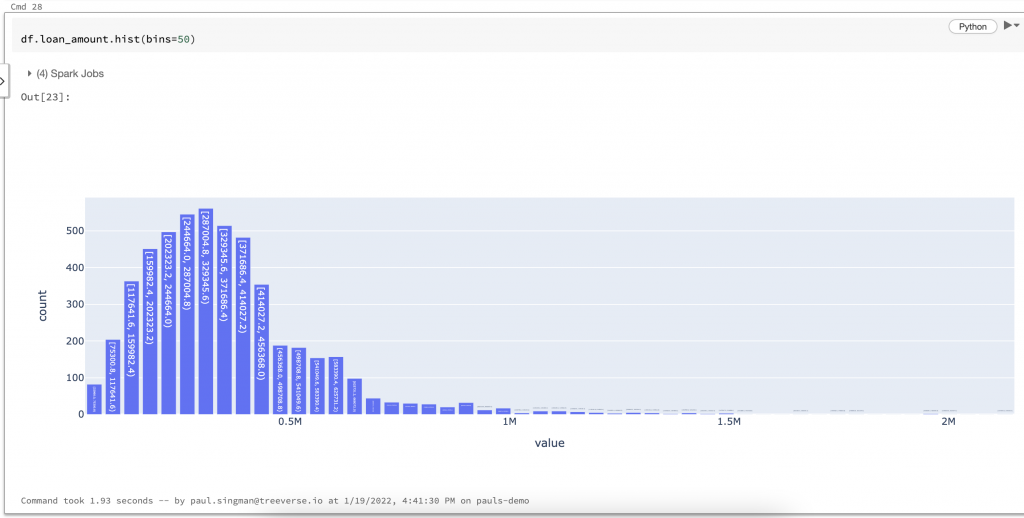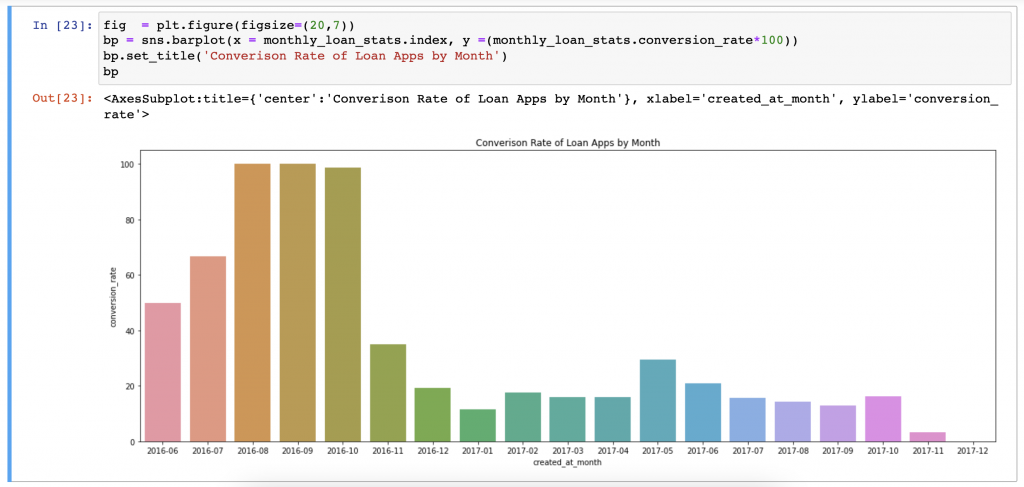# How Easy It Is to Re-use Old Pandas Code in Spark 3.2?

## In October, it was announced that the Pandas API was being integrated with Spark. This is particularly exciting news for a Pandas-baby like myself, whose first exposure to data exploration involved following tutorials using Jupyter notebooks and Pandas DataFrames.

Spark 3.2 has been out for several months now and a curiosity has been building inside me — how easy it is to take existing pandas code and copy it as is into Spark? Will it run without any errors or are there small last-mile API differences still being worked out?

You’re in luck if you have the same curiosity, because this is exactly what we’re going to test in this article. We’ll do so by digging up one of my favorite Pandas-based data exploration notebooks from the past and seeing if it can run in Spark (with all the distributed benefits Spark offers).

# Why It Matters

If my old code runs without requiring any changes, that would be amazing. If errors start getting raised, that’s okay, but it does give a sense of how easy it’ll be to port over Pandas code you wrote a couple of years ago.

If you want to see the original jupyter notebook in its entirety, click here. If you want to see the Databricks notebook with the new Pandas-on-Spark code, click here.

If you’re interested in a more detailed walk-through of what worked and what did not, how best to ingest data with what data types, stay along for the ride!

# Reading Data Into a DataFrame

There are two ways to start using Pandas-on-Spark. The first is to take an existing Spark DataFrame and calling the `to_pandas_on_spark()` method. The second imports directly to a DataFrame by reading from a file object with the same methods used in Pandas. The only difference is we import the pandas library from pyspark like so:

This is the Pandas code I wrote several years ago to read in a CSV file of loan data that contains 4 columns and about 5k rows. Does it run the same with Pandas-on-Spark?

Sadly, no. We get the following error:

Let’s try turning the parse_dates parameter to False and re-running. Luckily this quick fix works as you can see below.

Wonderful. We have a DataFrame and all of the data types look as expected. So it’s easier to follow along with the analysis, here a what the DataFrame looks like:

If you look at the original notebook, you’ll see I next checked for outlier datapoints and removed them. After that I explored behavior around the funding of loans: What percentage of loans created get funded? And how long does the process (called conversion) take?

Note that a loan with a date in the funded_at columns means it was funded, otherwise it is “None”. The following analysis shows how I answered these questions.

# Histogram Plots and Removing Outliers

How should we check for outliers in the loan_amount column? Create a simple histogram of course! This is what notebooks are great at after all.

Below are the histograms from Pandas (left) and Pandas-on-Spark (right) side-by-side. They both look equally unappealing due to some outlier loan amounts.

I used the following code to remove the bottom 0.5% and top 0.1% of loans to remove the outliers. The original code ran smoothly without any changes.

`q999 = df.loan_amount.quantile(0.999)q005 = df.loan_amount.quantile(0.005)# only include loan amounts less than or equal to  99.9 quantiledf = df[df.loan_amount <= q999]# only include loan amounts greater than or equal to 0.5 quantiledf = df[df.loan_amount >= q005]`

We get a much nicer histogram after removing these outliers! It’s nice to see the inline plotting look so good in the Databricks notebook with some interactive features as well.Loan Amount with outliers removed. Much nicer!

# Calculating Conversion Over Time

Now it’s time to get to the meat of the analysis and answer the big questions: How long does it take for a loan to go from “created” to funded?” and What percentage of loans get funded?

We start by subtracting the two date columns to calculate a time_to_conversion metric. In Pandas this looked like:

`# Let's create a time_to_conversion fieldloans_df['time_to_conversion'] = (loans_df.funded_at - loans_df.created_at).dt.days`

Sadly, the same doesn’t work in Pandas-on-Spark, where instead get the following warning.

/databricks/spark/python/pyspark/pandas/data_type_ops/datetime_ops.py:61: UserWarning: Note that there is a behavior difference of timestamp subtraction. The timestamp subtraction returns an integer in seconds, whereas pandas returns ‘timedelta64[ns]’.

This is caused by a known issue in Koalas & Pandas-on-Spark, where date subtractions return a different type of object as the warning informs. I was able to work around this by manually converting from seconds back to days after subtracting the dates.

`df['time_to_conversion'] = (df.funded_at - df.created_at) / (60*60*24)`

Great, now we have a metric that calculates how long it takes for a loan to get funded. What’s important is to understand how this metric has changed over time.

To do this, I decided to group loans into monthly cohorts and then plot the following metrics month:

• No. of loan applications
• No. of loans funded
• Percent of loans converted
• Average time to conversion

The Pandas code and one of the resulting graphs showing Average Conversion Rate by Month looked like this:Seaborn chart of monthly Coversion Rate.

From the chart, we get a much clearer idea how frequently loans were funded at the end of 2016 compared to the more recent months in this dataset. More important to this article though, does the same run in Spark? Sadly, again no.

There ended up being two small issues to work through before I could re-create the Conversion Rate of Loan Apps by Month plot.

1. The `to_period` method used in line 13 of the Pandas code doesn’t exist yet in Pandas-on-Spark.
2. The way we pass a column from the loans DataFrame to Seaborn’s barplot method is slightly different.

Both error messages are shown below:

The first I was able to fix by instead using string manipulation of the `created_at` date column to arrive at the same result. The second I found a simple fix passing the column as NumPy arrays from a StackOverflow answer.

The updated code is shown below along with the final plot!

# Wrapping Up

Overall, it wasn’t too difficult to get my Pandas code to work with Pandas-on-Spark. However, it was far from the errorless experience I was hoping for.

If it is the case that you have a dataset you are analyzing with Pandas and it grows too large to fit in your machine’s memory, running it in a Spark environment is likely still the easiest way to scale. Try it yourself and let me know how it goes!